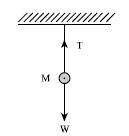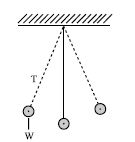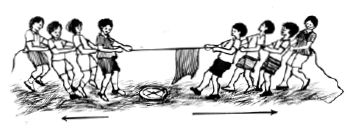Physics

# Explain Balanced and Unbalanced Forces

When two or more forces acting on an object produce zero resultant force, i.e. the object has no acceleration; we say that the forces are balanced. The forces that produce this balanced condition are called balanced forces.Fig (a)                           Fig (b)

It is seen in figure (a) that an object is suspended with a thread. The force of attraction of earth on the object i.e. the weight of the object W is acting vertically downward. The tension of the thread T is acting vertically upward. Here the two forces are equal in magnitude but opposite in direction thus canceling each other’s action and producing a balanced condition.

Only the attractive force of the earth i.e. the force of gravity will act on the object if the thread is cut. Then the object will fall down with acceleration due to gravity. Here, the force of gravity or weight of the object is the unbalanced force. If the body is displaced slightly along one side, the tension of the thread T and the weight W will not be in a straight line. Then a resultant force will act on the body without creating balanced condition. Due to this, the body will oscillate. This is an example of unbalanced force.

Another example of balanced and unbalanced forces can be seen in the game of tug-of-war competition. In this game, a handkerchief is tied to the center of the rope. In this competition equal numbers of competitors pull on the rope in two sides and try to move the handkerchief to their side. If the handkerchief does not move then it is understood that either the team are applying equal amount of force and the rope or the handkerchief is in balanced condition. Here, forces applied by the two teams are balanced forces. If one team applies more force than the other, then the resultant force will act along them thus creating unbalanced force and the handkerchief will move to their side Then this team will be declared as the winning team in the competition.Fig: Tug-of-war (unbalanced force)